# Structural and kinematic modeling of porcine aortic valve### Description

• In this exercise, you will learn how to perform strain analysis on a finite element model of a square section of a heart valve cusp under a simulated biaxial stretch by utilizing the displacements of surface markers.
• Realistic collagen fiber architecture in the finite element will be fitted to fiber angle measurements
• This will require you to create a simple material coordinate model to mathematically define the fiber structure.

• A continuous strain map will be calculated from the displacement of surface markers on the stretched valve.
• A finite element model of the initially undeformed square section of valve will be fitted to the displacements of the markers to calculate a deformed configuration and the resulting strain.
• These techniques for analyzing valve mechanics can be useful in the design of artificial or tissue engineered replacement valves.

### Modelling the collagen fiber structure

#### Defining a material coordinate system

• One of the key determinants of biological tissue mechanics is its underlying microstructure; in the case of a heart valve, the orientation of collagen fibers throughout the tissue must be considered for accurate/realistic mechanical analysis. Before we can describe the collagen fiber structure in a finite element, we need a means to calculate the orientation of fibers in three dimensional Euclidian space (the rectangular Cartesian global coordinate system). We can accomplish this by defining a local orthogonal coordinate system that is aligned with the local collagen fiber; this is called the fiber or material coordinate system, and calculated vector/tensor quantities like stresses and strains are resolved with respect to this coordinate system.

• A material coordinate model in Continuity constructs the mathematical relations (i.e. transformations) between coordinate systems, ultimately arriving at the transformation/rotation from the global rectangular Cartesian coordinate system (Y in the figure below, Y in the code) to the local fiber/material coordinate system (X in the figure below, Matl in the code). This transformation is stored as a variable named dY_dMatl (the matrix of derivatives of the Y coordinates with respect to the Matl coordinates) in the code and is the final output of the model. An intuitive way to describe the orientation is with a fiber angle that rotates the material coordinate system with respect to the local finite element coordinate system (ksi in the figure below, Xi in the code).

• The world coordinate system (theta in the figure below, X in the code) can be used to define curvilinear coordinate systems such as cylindrical, spheroidal, or prolate spheroidal for anatomical structures that naturally assume these shapes (blood vessel, eyeball, or heart, respectively). For the purposes of this exercise, we use a simple rectangular Cartesian coordinate system; in this case, Y = theta in the figure, Y=X in the code.

• First, we will look at an example of an existing material coordinate model to understand how it works.
• Launch the Continuity 6.4 Client
• Open the Continuity database: File→Library→Search…

• Search for Title containing matcoordstandard.

• Continuity’s standard material coordinate model (Costa, 1996):

•• Right-click on the search result, Load, and Reset (without save) then proceed.

• Open the material coordinate model editor: Mesh→Edit→Material Coordinates…

• View the mathematical expressions defining Continuity’s standard material coordinate transformation. This transformation is defined by the variable dY_dMatl that rotates the rectangular Cartesian global coordinates to the local fiber/material coordinates.

• All coordinate models are built from the independent tensor variables:
• X – the vector of undeformed world cooordinates

• dX_dXi – the tensor of undeformed world coordinate derivatives with respect to local finite element coordinates

• d2X_dXi2– the tensor of undeformed world coordinate second derivatives with respect to local finite element coordinates

• Y – the vector of undeformed rectangular Cartesian coordinates

• dY_dXi – the tensor of undeformed rectangular Cartesian coordinate derivatives with respect to local finite element coordinates

• dY2_dXi2 – the tensor of undeformed rectangular Cartesian coordinate second derivatives with respect to local finite element coordinates

• From these independent quantities and other user-defined dependent quantities, transformations from the Continuity-defined world Xrectangular Cartesian Y, and finite element Xi coordinates to the user-defined material coordinate system can be calculated by multiplying different combinations of these transformations (the tensors of derivatives) together to arrive at dY_dMatl by the chain rule.

### Initialize the valve model

• Reset the current model
• Lose all data for Open Mesh, Client, Server
• Load the model: File→Load Model… or click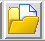• Download the model Valve_initial.cont6 and load it from the file browser.

• Click Open.

• The mesh data is loaded to the Continuity client.
• Send the mesh data stored in the Continuity client to the Continuity server: File→Send or click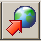• The server must be updated every time changes are made to the mesh before performing any calculations.

• Calculate the mesh geometry: Mesh→Calculate Mesh… or click• Use the default settings.
• Click OK.

• Render the undeformed mesh: Mesh→Render→Elements… or click• To render the element lines, select the lines and undeformed radio buttons.

• Click Render.

• To render an element surface, select the surfaces and undeformed radio buttons.

• Choose an Xi plane (12 or 3) to render.

• Enter an Xi Location between 0.0 and 1.0 to render a surface at this constant Xi coordinate.

• Click Render.

• The result:
•The finite element is three dimensional even though we will model the fiber structure and strain in two dimensions (Xi1 and Xi2); there will be no variation of these quantities in the Xi3 direction.

#### Create a material coordinate system model

• Now, we will create our own transformation law for dY_dMatl. This model is initialized with an empty transformation law.

• Open the material coordinate model editor: Mesh→Edit→Material Coordinates…

• Right click the root component MatCoordModel and select add variable inside.

• Select the new variable (evaluated1), rename it to dY_dMatl, and write an expression (Sympy syntax) for dY_dMatl that rotates the Y coordinate system to the Matl coordinate system by the local fiber angle.

•• The variable for the fiber angle will need to be entered as a parameter since it varies in the mesh domain. To input variables as parameters in the equations, enclose the variable name with < and > (e.g. <fiber_angle>). The SymPy to C parser interprets these variables as parameters.

• A new parameter fiber_angle will populate the list. Set For default values use Fiber Angle. This sets the value of the local fiber angle to that interpolated within the finite element as defined by the nodal parameters and basis functions under the Fiber Angle tab of the Node Form.

•• Now we are ready to dynamically compile the model equations written in SymPy to faster C code and produce the binary file that Continuity will use to implement the model in its calculations.

• Click Compile… and select Compile Code as C: Double Precision.

• Known compile issues by platform (release 7113):

• Windows 7 or Vista: If your copy of Continuity is installed in the default Programs Folder, it is likely that you do not have permission to write/modify its contents, even if you are the admin user of your own computer. Try running the Continuity application shortcut as Administrator or modifying the folder’s security properties to grant adequate privileges to create the new compiled files. A confirmed fix is to reinstall Continuity in a directory other than the Programs Folder.

• Mac OSX 10.6: Users receive the following error message:
• ```         <type 'exceptions.AttributeError'>
MatCoordBuild instance has no attribute 'src_prefix'
```

First, make sure that XCode is installed on your Mac. Second, Continuity has to be setup to compile (it’s not automated yet) with this script on the Continuity Plugins page; this requirement isn’t yet automated. After unzipping the file, double-click it and select the Continuity directory to configure (e.g., /Applications/continuity). A message window will pop up confirming that the build environment has been set correctly. For more details, see the page Compiling models with Continiuty for Macs.

• If compilation was successful, Export your material coordinate model to a text file which can later be read into different Continuity models.

• To verify that the material coordinate model works, we will render the material coordinate axes: Mesh→Render→Fibers… or click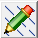• Set the Xi3 Locations to 0.0.

• Specify a vector Length. You can still edit the length after rendering.

• Specify the number of material vectors to render per element in each direction. Render a dense field of about 25 vectors in each direction.
• Under the Vector section, check all three material axes. Select Render axes separately. The first material axis is aligned with the long axis of the fiber.

• Choose how to color the vectors.
• Click OK to render the fibers.At this point, we don’t have any fiber angles included in the model; all angles are zero. The fiber field should look like a regular grid of t’s. Note that the angle of the first material axis is zero with respect to the Xi1direction of the finite element coordinate system and the X direction (red global axis) of the rectangular Cartesian global coordinate system.

(If you want to check the rendering for a nonzero fiber angle, set the <fiber_angle> parameter to Value, and enter a test angle (in radians). You don’t have to recompile the model to change parameters.)

#### Fitting a collagen fiber field from experimental data to a finite element mesh

• The finite element method enables us to interpolate any scalar, vector, or tensor quantity on the surface (2D) or within the volume (3D) of a mesh. In order to realistically model the collagen fiber architecture of a heart valve, we will interpolate a scalar field of fiber angles over the element which best fits an experimentally measured fiber angle field.
##### Experimental measurements

• The paper (Joyce, 2008) provides the following figure from which we can directly measure fiber angles:

•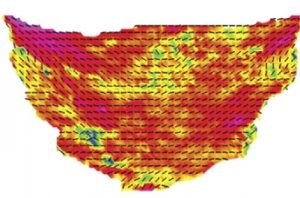The figure below illustrates an example layout of the finite element mesh and a grid of measurement points over a square patch of the valve in the above figure. In this example, the angle of the vector directly above each measurement number was measured with respect to the horizontal axis using the Angle Tool in id=ie7&rls=com.microsoft:en-us:IE-Address&ie=&oe= ImageJ and was bounded to lie between +90 and -90 degrees.

•Enter your own measurements of spatial coordinates and fiber angle (converted to radians) over a patch of valve into a spreadsheet in the format of this example data file:

•The column header coord_1_val is the x value of the data point, coord_2_val is the y value of the data point, etc. Notice that coord_3_val is slightly offset from zero so that it resides within the 3D finite element. angle_val is the fiber angle measurement at each point in radians. *_*_weight applies a weight to each data point to be used in the subsequent fitting process; this is normally defaulted to a value of 1. Save this spreadsheet as a tab-delimited text file. We are now ready fit this data into our finite element model.

##### Fitting procedure

• Download the model Valve_angle_prefit.cont6 and open it in Continuity.

• Change the material coordinate model to the one you created above by importing the model you previously saved.

• Load the angle data:
• The following data table will appear:• Click Import/Export/Graph. A second data table will appear:• Open the tab-delimited text file where the angle measurements are saved (sample data file). The table will become populated by the contents of the file. Close and update form.

• The first data table will become populated by your measurements. Click OK.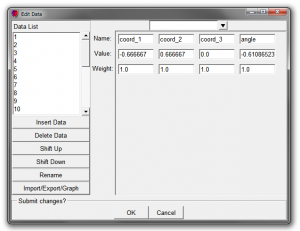• Send the newly updated data to the server: File→Send or click• Save the model or click.

•Before fitting, make sure you have a basis function specified for the Fiber Angle field in the nodes form. During the fit, Continuity will solve for the nodal parameters (values and derivatives) of the basis functions so that the least amount of error between the interpolated field and the actual data is obtained. If a basis function is not selected, Continuity doesn’t know that it is a field to be fitted. Select the appropriate bases (Linear-Linear-Linear Lagrange or Cubic-Cubic-Linear Hermite) judging by a visual estimate of the amount of fiber angle variation from your selected patch of measurements.

• {!} A known issue exists in Continuity where two forms must be opened and closed to correctly initialize a variable; failure to do this could result in an error in an attempted fit.

• Open and close the following forms:

• SendCalculate meshSave.

• Open the Fit Data form:

• Under the Coordinates tab, ensure that the correct data column corresponds to the correct coordinate• Under the Fibers tab, select angle for Fiber Angle.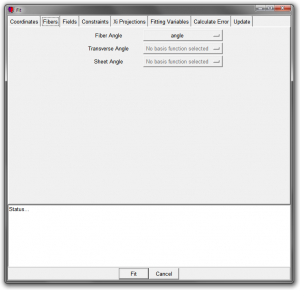• Under the Constraints tab, check ‘Also apply constraints to initial nodal degrees of freedom before fit’.• Under the Fitting Variables tab, check only Fiber Angle (we are not yet doing a geometric fit of the mesh).• Click Fit. Note the resulting fitting error. The error has the same units as the fitted data (radians in this case). The optimal error is one that is on the same order of magnitude as the measurement error in the data; larger errors indicate that a more accurate fit can be obtained, while smaller errors indicate that unwanted noise has been fitted.

• Render the fitted fiber field as done previously: Mesh→Render→Fibers… or click• If you set the Fiber Angle parameter to value of a constant angle in your material coordinate model, you need to change it to For default values use Fiber Angle to use the fitted angle field.

• The sample data produces the following fitted field:
•• Visually compare the fiber directions as rendered in the model to the original figure from which the angle measurements were taken; the vector orientations should be close at the measurement points (blue circles in the figure above) if the fit was good.

### Modeling valve kinematics

Now we can model valve kinematics with the fitted fiber field. First, we need a deformation to simulate.

#### Generating artificial deformation data

Using MatlabExcel, or graphing paper and a calculator, generate an nxn grid of (at least 5x5) evenly spaced points over the finite element to serve as the artificial surface markers in the undeformed configuration. Make sure the points are sufficiently close to the edges of the element so that the deformation is well-defined over the entire element. Apply a homogeneous deformation in the form of y = F*Y, where y and Y are (n^2)x2 matrices of the 2D marker coordinates in the deformed and undeformed configurations, respectively, and F is a 2x2 deformation or displacement gradient tensor. Consider a physiologically relevant magnitude of deformation. Add some additional noise to the marker coordinates to make the deformation more nonuniform.#### Locate material points on the element (Calculate the Xi coordinates of the markers)

An underlying assumption of our kinematic simulation is that the surface markers remain at the same material points on the valve/element throughout the deformation, and a particular material point retains the same relative position to its neighbors in the undeformed and deformed states . We can naturally implement this assumption using the finite element coordinate system Xi which remains normalized ([0 1]) in the deformation. This allows us to fit a deformed element such that the material points of the markers in Xi coordinates in the undeformed state are displaced to match the positions of the deformed markers in the rectangular global Cartesian coordinate system Y (remember that the geometry of the element in Y coordinates is parameterized as a function of the Xi coordinates in the undeformed and deformed states).

We can calculate the Xi coordinates of the markers in the undeformed state in Continuity by projecting the data points onto the surface of the 2D element:

• Load the undeformed marker coordinates.
• Follow the same steps as when adding the fiber angle data.
• The data form for the undeformed coordinates should use the same format as fiber angle data form; delete the columns for angle_val and angle_weight.

• Calculate the Xi coordinates:
• Under the Xi Projections tab, check Display resulting Xi table without fitting• Click Fit.

• A table with the results will pop up:• Save the table to a file called list_xis.txt.

• Examine list_xis.txt in a spreadsheet document:

•• The contents of the file include the Xi coordinates of the markers in the first three columns. Remember that the Xicoordinates are normalized, ranging from 0 to 1 in each direction. Distance measures the length of the projection; this is the distance of the data point in space to its location on the element surface (in the case of a 3D element, points that lie in the volume of the element have a projection distance of 0). Dot product measures the angle at which the projection line makes with the surface normal at the projection point. Element lists which element in which the projection lies. Data identifies the data point.

#### Fit a deformed element

Now we are ready to fit a deformed geometry.

• Load the artifcial deformation data you generated into the data form in the same way as you entered the fiber angle data:
• Import/Export/GraphOpenClose and update form, etc.

• Send, calculate, and save your mesh:
• File→Send or click• Mesh→Calculate Mesh… or click• Save→Save model as… or click• Save under a filename like Valve_def_prefit.cont6.

• It’s good practice to perform these three steps after making any changes to the mesh and before any major calculation., especially Send and Calculate mesh. Otherwise, you will get errors due to incorrect initialization of the sizes of the arrays that Continuity uses. This accounts for many known bugs.

• Before we continue with the fit, we need to convert the element from trilinear basis functions to bicubic-linear basis functions:

• In defining the initial mesh, you should have chosen a trilinear basis in the nodes form since you only specify the coordinate values of the nodes and not their derivatives. However, to model a deformed configuration, we need higher order bases to capture the changes in curvature of the element. The bases should be converted to a bicubic-linear basis (cubic in the , but the derivatives need to be calculated first for the cubic geometric interpolation to be mathematically equal to the linear interpolation.

• Refining a mesh in Continuity calculates these derivatives for free:

• Enter the settings as illustrated below and click OK.:

•• The derivatives have now been calculated.
• Add tricubic basis functions if not already:
• Select Choose Hermit Basis Function3DCubic-Cubic-Linear.

• Click Add Cubic-Cubic-Linear.

• Click OK.

• Change the nodal basis functions to Cubic-Cubic-Linear.
• Select Cubic-Cubic-Linear for all three coordinates

• Click OK.

• SendCalculate Mesh, and Save.

•First, we should save the nodes form of the undeformed mesh with the fitted fiber angles to a text file so we can use it later as our reference state before deformation:

• Click Import/Export/Graph.

• Save the nodes form as nodes_undef.txt.

• Second, we can render the deformed data so we know what kind of deformation we should expect:
• Use the default settings and click OK.

• Now we will perform the actual fit.
• Under the Constraints tab, check ‘Also apply constraints to initial nodal degrees of freedom before fit’.

• Under the Fitting Variables tab, check Coordinate 1 and Coordinate 2Uncheck Coordinate 3.

• Under the Xi projections tab, set Get xi coordinates to from table.

•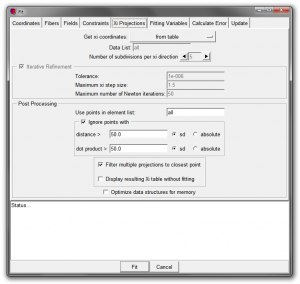• Click Fit.

• Select the file ‘list_xis.txt’ with the previously calculated Xi coordinates of the surface markers.

• Note the fitting error. The deformed mesh has now been fitted.
• Click Send and Calculate mesh.

• Save the model under a new file name such as Valve_def_postfit.cont6.

• Render the result
• Render→Elements… or click• Select the deformed radio button.

• Click Render.

• Your fitted mesh should look like this:
•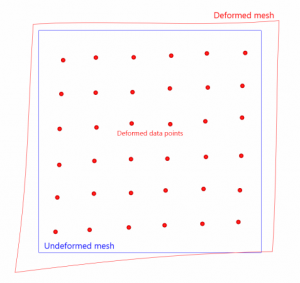• Check that the deformed mesh looks reasonable. If your data points were not close to the edges of the mesh, there may be bad behavior at the edges, in which case you may need to apply fitting constraints or smoothing weights. This is a more advanced topic and will not be explained here.

Now we will render the strain map of the deformation.

• If not using the example model, Save your model under a different file name.

• Add the biomechanics module by clickingand checking biomechanics.

• We need to define a constitutive model for the purpose of calculating kinematics (even though we will not be analyzing stress, the equations for strain are defined in the constitutive models).

• Similar to the material coordinate model, write your own equations to compute Lagrangian strains E (hint: first write F as an expression of the default Independent Variables)

• Compile the model as C Double Precision.

• Under the Submit tab, click Submit.

• Send and Calculate mesh.

• Now we need to define the undeformed and deformed configurations of our mesh. (The following steps have already been done in the example model).
• Deformed configuration

• This step takes the nodal parameters defined in the Nodes form (Mesh→Edit→Nodes…) and loads them into the Initial Conditions tab in the Boundary Conditions form (Biomechanics→Edit→Boundary Conditions). Continuity takes meshes defined in the Nodes form as the undeformed configuration and meshes defined in the Initial Conditions tab as the deformed configuration. In our case, the result of the geometric fit has been saved in the Nodesform, but we need to transfer it to the Initial Conditions form so that Continuity sees this as the deformed mesh.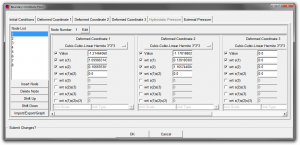• Verify that the nodal parameters are the result of the previous fit.
• Undeformed configuration

• Click Import/Export/Graph.

• File→Open the undeformed nodes file you saved earlier (nodes_undef.txt).

• Close and update form.

• Click OK.

• SendCalculate mesh, and Save.

• Make sure you have a basis function specified for Field Variable 1 under the Field Vector 1 tab in the Nodes form.

• Render the fiber (xx) component of the Lagrangian Green’s Strain Tensor:

• Enter the form as illustrated below:
•• Your strain map should look like this:
••There is a known issue in version 7113 at this step. Continuity crashes upon selecting Render→Surface. The cause of this is related to the basis function used for the Field Variable 1 in the Nodes form which Continuity uses for its interpolation of the strain map. If you experience this crash, try the following:

• First, make sure that your basis functions for the undeformed and deformed nodes are the same.
• Save your model, exit Continuity and reopen it.

• In the Nodes form, if there is a basis function specified for Field Variable 1 (due to a previous attempt to render the strain), deselect it so that Field Variable 1 has no basis and all its nodal parameters are grayed out.

• Under View→Edit Dimensions…, you will probably find that there is a dimension error since you have removed one of the Geometric Variables by removing its basis. Click Apply Marked Recommendations to correctly set the size to 4.

• Send and Calculate Mesh.

• Try Biomechanics→Render→Surface… again.
• Continuity will automatically add a basis function to Field Variable 1

• If Continuity does NOT crash and the render form appears, close the form and change the basis function for Field Variable 1 to Linear-Linear. If the render form appears again, the rendering should work.

• If Continuity does crash at this point, close and reload your model. This time set the basis function for Field Variable 1 to Linear-Linear before Biomechanics→Render→Surface…; check dimensions, Send and Calculate Mesh, and attempt to render again.

• In Open Mesh Controls (click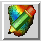), check if the range of the strain in the colormap is reasonable; these are the maximum and minimum values of strain. You can also change the Color Scheme• Save the model as valve_final.cont6.